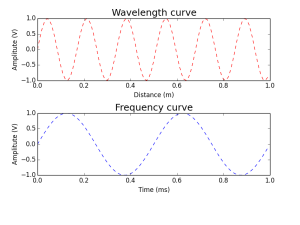# Comparison between Frequecy, Wavelength & Time period

FREQUENCY, WAVELENGTH & TIME PERIOD

In general, frequency, wavelength & time period are quite confusing parameters. Indeed, these three are entirely different in nature.

FREQUENCY

Frequency is the number of occurrences of a repeating event per unit time. Unit of frequency is hertz(hz).

WAVELENGTH

Wavelength is the distance between identical points in the adjacent cycles of a waveform signal propagated in space or along a wire. Unit of wavelength is meter(m).

TIME PERIOD

The time taken to complete one full cycle is said to be time period. Unit of time period is meter/second (ms).

EXAMPLE

Lets take a signal at 2 kHz. Then the period is 0.5 ms. To plot this as a function of time.If a sound wave is travelling in space with a speed of 340 m/s then the wavelength is the distance the sound will travel in a period 340 * 0.5m = 17 cm. (i.e) 0.17m

PYTHON CODE

import numpy as n

import matplotlib.pyplot as p

f = 2

y = .17

d =n.arange(0,1,0.01)

t=n.arange(0,1,0.01)

wavelength =n.sin(2*n.pi/y*d)

frequency =n.sin(2*n.pi*f*t)

p.subplot(211)

p.plot(t, wavelength,’r–‘)

p.xlabel(‘Distance (m)’)

p.ylabel(‘Amplitute (V)’)

p.title(‘Wavelength curve’, fontsize=18)

p.subplot(212)

p.plot(d,frequency,’b–‘)

p.xlabel(‘Time (ms)’)

p.ylabel(‘Amplitute (V)’)

p.title(‘Frequency curve’, fontsize=18)

p.show(block=False)

WAVEFORM